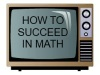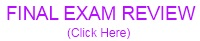CALCULUS FOR BUSINESS/ECONOMICSSoftware Install (this is the player that will allow you to watch recorded webex class sessions): (Click Here)Note: When playing the webex movies, you will need to close the participants and table of contents window (click the x in the upper right). If you want to skip ahead, you probably will want to pause it first, move it to where you want and then click the play. Section A.4 - Factoring Polynomials: (Webex Video)   (YouTube Video) Problems (pdf): (Click Here) Problems (powerpoint): (Click Here) PDF Pages: (Page 1)   (Page 2)   (Page 3)   (Page 4)   (Page 5)   Section 1.5 - Limits: (YouTube Video) Problems (pdf): (Click Here) Problems (powerpoint): (Click Here) PDF Pages: (Page 1)   (Page 2)   (Page 3)   (Page 4)   (Page 5)   Section 1.6 - Continuity: (YouTube Video) Problems (pdf): (Click Here) Problems (powerpoint): (Click Here) PDF Pages: (Page 1)   (Page 2)   (Page 3)   (Page 4)   Section 2.1 - The Derivative and the Slope of a Graph: (Webex Video)   (YouTube Video) Problems (pdf): (Click Here) Problems (powerpoint): (Click Here) PDF Pages: (Page 1)   (Page 2)   (Page 3)   (Page 4)   (Page 5)   (Page 6)   Section 2.2 - Some Rules of Differentiation: (Webex Video)   (YouTube Video) Problems (pdf): (Click Here) Problems (powerpoint): (Click Here) PDF Pages: (Page 1)   (Page 2)   (Page 3)   (Page 4)   (Page 5)   (Page 6)   Section 2.3 - Rates of Change: Velocity and Marginals : (YouTube Video) Problems (pdf): (Click Here) Problems (powerpoint): (Click Here) PDF Pages: (Page 1)   (Page 2)   Section 2.4 - The Product and Quotient Rules (Webex Video)    (YouTube Video) Problems (pdf): (Click Here) Problems (powerpoint): (Click Here) PDF Pages: (Page 1)   (Page 2)   (Page 3)   (Page 4)   (Page 5)   (Page 6)   (Page 7)   (Page 8)   (Page 9)   Section 2.5 - The Chain Rule (Webex Video)    (YouTube Video) Problems (pdf): (Click Here) Problems (powerpoint): (Click Here) PDF Pages: (Page 1)   (Page 2)   (Page 3)   (Page 4)   (Page 5)   (Page 6)   Section 2.6 - Higher-Order Derivatives (Webex Video)    (YouTube Video) Problems (pdf): (Click Here) Problems (powerpoint): (Click Here) PDF Pages: (Page 1)   (Page 2)   Section 2.7 - Implicit Differentiation (Webex Video)    (YouTube Video) Problems (pdf): (Click Here) Problems (powerpoint): (Click Here) PDF Pages: (Page 1)   (Page 2)   (Page 3)   (Page 4)   (Page 5)   Section 2.8 - Related Rates (Webex Video)    (YouTube Video) Problems (pdf): (Click Here) Problems (powerpoint): (Click Here) PDF Pages: (Page 1)   (Page 2)   (Page 3)   Chapter 1 and 2 Test Review Test Review: (pdf) YouTube Explanations: (#01)    (#02)    (#03)    (#04)    (#05)    (#06)    (#07)    (#08)   (#09)   (#10)   (#11)   Section 3.1 - Increasing and Decreasing Functions (Webex Video)    (YouTube Video) Problems (pdf): (Click Here) Problems (powerpoint): (Click Here) PDF Pages: (Page 1)   (Page 2)   (Page 3)   (Page 4)   (Page 5)   (Page 6)   (Page 7)   (Page 8)   Section 3.2 - Extrema and the First-Derivative Test (Webex Video)    (YouTube Video) Problems (pdf): (Click Here) Problems (powerpoint): (Click Here) PDF Pages: (Page 1)   (Page 2)   (Page 3)   (Page 4)   (Page 5)   (Page 6)   (Page 7)   (Page 8)   (Page 9)   (Page 10)   (Page 11)   (Page 12)   Section 3.3 - Concavity and the Second Derivative Test (Webex Video)    (YouTube Video) Problems (pdf): (Click Here) Problems (powerpoint): (Click Here) PDF Pages: (Page 1)   (Page 2)   (Page 3)   (Page 4)   (Page 5)   (Page 6)   Section 3.4 - Optimization Problems (Webex Video)    (YouTube Video) Problems (pdf): (Click Here) Problems (powerpoint): (Click Here) PDF Pages: (Page 1)   (Page 2)   (Page 3)   (Page 4)   Section 3.5 - Business, Economics, and Medical Applications (Webex Video)    (YouTube Video) Problems (pdf): (Click Here) Problems (powerpoint): (Click Here) PDF Pages: (Page 1)   (Page 2)   (Page 3)   (Page 4)   (Page 5)   (Page 6)   Section 3.6 - Asymptotes (Webex Video)    (YouTube Video) Problems (pdf): (Click Here) Problems (powerpoint): (Click Here) PDF Pages: (Page 1)   (Page 2)   (Page 3)   (Page 4)   (Page 5)   Section 3.7 - Curve Sketching: A Summary (Webex Video)    (YouTube Video) Problems (pdf): (Click Here) Problems (powerpoint): (Click Here) PDF Pages: (Page 1)   (Page 2)   (Page 3)   (Page 4)   (Page 5)   (Page 6)   (Page 7)   Section 3.8 - Differentials and Marginal Analysis (Webex Video)    (YouTube Video) Problems (pdf): (Click Here) Problems (powerpoint): (Click Here) PDF Pages: (Page 1)   (Page 2)   (Page 3)   Chapter 3 Test Review Test Review: (pdf) YouTube Explanations: (#01)    (#02)    (#03)    (#04)    (#05)    (#06)    (#07)    (#08)   (#09)   (#10)   Section 4.1 - Exponential Functions (Webex Video)    (YouTube Video) Problems (pdf): (Click Here) Problems (powerpoint): (Click Here) PDF Pages: (Page 1)   (Page 2)   (Page 3)   Section 4.2 - Natural Exponential Functions (Webex Video)    (YouTube Video) Problems (pdf): (Click Here) Problems (powerpoint): (Click Here) PDF Pages: (Page 1)   (Page 2)   (Page 3)   (Page 4)   (Page 5)   Section 4.3 - Derivatives of Exponential Functions (Webex Video)    (YouTube Video) Problems (pdf): (Click Here) Problems (powerpoint): (Click Here) PDF Pages: (Page 1)   (Page 2)   (Page 3)   (Page 4)   (Page 5)   (Page 6)   (Page 7)   (Page 8)   (Page 9)   (Page 10)   Section 4.4 - Logarithmic Functions (Webex Video)    (YouTube Video) Problems (pdf): (Click Here) Problems (powerpoint): (Click Here) PDF Pages: (Page 1)   (Page 2)   (Page 3)   (Page 4)   Section 4.5 - Derivatives of Logarithmic Functions (Webex Video)    (YouTube Video) Problems (pdf): (Click Here) Problems (powerpoint): (Click Here) PDF Pages: (Page 1)   (Page 2)   (Page 3)   (Page 4)   (Page 5)   (Page 6)   (Page 7)   (Page 8)   (Page 9)   Chapter 4 Test Review Test Review: (pdf) YouTube Explanations: (#01)    (#02)    (#03)    (#04)    (#05)    (#06)    (#07)    (#08)   (#09)   (#10)   Section 5.1 - Antiderivatives and Indefinite Integrals (Webex Video)    (YouTube Video) Problems (pdf): (Click Here) Problems (powerpoint): (Click Here) PDF Pages: (Page 1)   (Page 2)   (Page 3)   (Page 4)   (Page 5)   Section 5.2 - Integration by Substitution and the General Power Rule (Webex Video)    (YouTube Video) Problems (pdf): (Click Here) Problems (powerpoint): (Click Here) PDF Pages: (Page 1)   (Page 2)   (Page 3)   (Page 4)   (Page 5)   Section 5.3 - Exponential and Logarithmic Integrals (Webex Video)    (YouTube Video) Problems (pdf): (Click Here) Problems (powerpoint): (Click Here) PDF Pages: (Page 1)   (Page 2)   (Page 3)   (Page 4)   (Page 5)   Section 5.4 - Area and the Fundamental Theorem of Calculus (Webex Video)    (YouTube Video) Problems (pdf): (Click Here) Problems (powerpoint): (Click Here) PDF Pages: (Page 1)   (Page 2)   (Page 3)   (Page 4)   (Page 5)   (Page 6)   (Page 7)   Section 5.5 - The Area of a Region Bounded by Two Graphs (Webex Video)    (YouTube Video) Problems (pdf): (Click Here) Problems (powerpoint): (Click Here) PDF Pages: (Page 1)   (Page 2)   (Page 3)   (Page 4)   (Page 5)   (Page 6)   (Page 7)   Chapter 5 Test Review Test Review: (pdf) YouTube Explanations: (#01)    (#02)    (#03)    (#04)    (#05)    (#06)    (#07)    (#08)   (#09)   (#10)